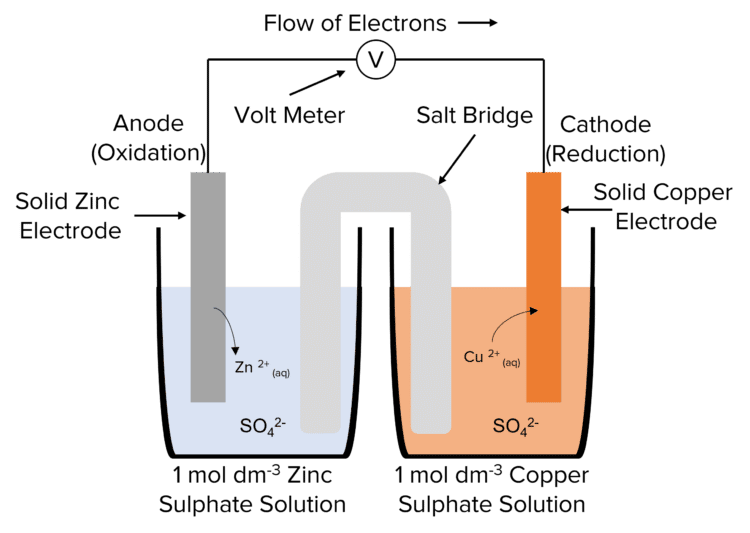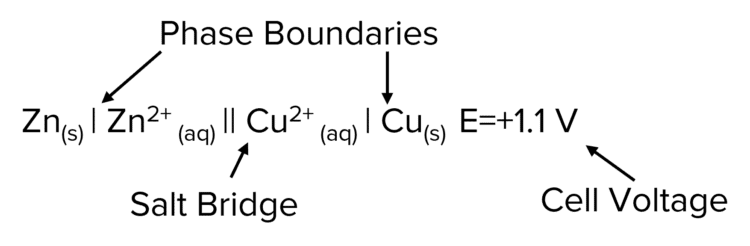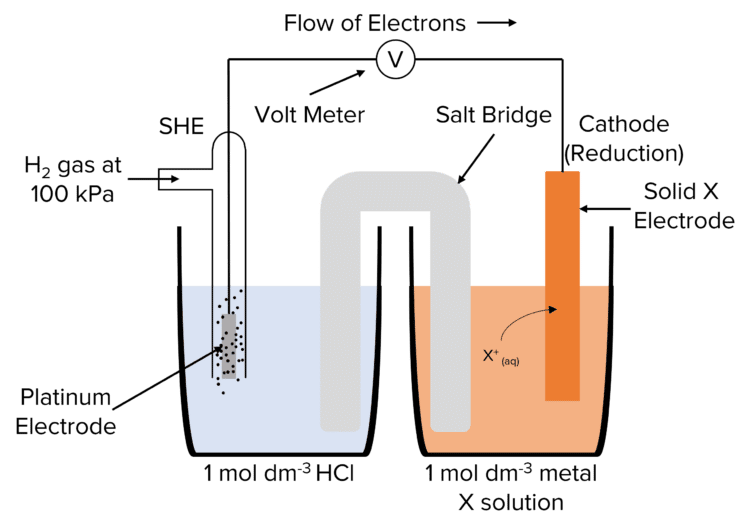# Electrochemical Cells

A LevelAQA

## Electrochemical Cells

Electrochemistry is a fantastically useful application of chemistry. Electrochemical cells use the electrons produced during redox reactions to power an electrical circuit. Electrochemical cells are the basis of modern batteries.

## The Structure of an Electrochemical Cell

Electrochemical cells are made up of two half-cells. Each half-cell is contains a solid metal electrode, immersed in a solution of an ionic compound containing that metal (called the electrolyte). The two half cells are though a circuit in which the electrons generated at the anode flow to the cathode. The circuit is completed by the salt bridge between the two half cells.The potential of a cell is generated by the redox processes taking place at the anode and cathode. Electrons are generated at the anode by the oxidation of the solid metal electrode. In this process, metal atoms lose electrons and dissolve into the solution as positive ions. These electrons are then transported to the cathode through a circuit. These electrons are then used to reduce positive metal ions at the cathode to produce solid metal atoms

As this process continues, the mass of the anode will decrease as the metal is oxidised into solution. The mass of the cathode will increase as positive metal ions are reduced to solid metal. These reactions will eventually stop as the reactants are used up. At this point, as no more electrons are produced to move around the circuit, the cell voltage drops to $0$

The voltage of the cell is usually read from a voltmeter. The voltmeter will have a high resistance. This prevents the voltmeter drawing current from the circuit, allowing the maximum cell potential to me measure.

The presence of the salt bridge will complete the circuit by allowing the negative ions of the solution to transfer between the two half cells. This allows the current to flow through the whole system. To allow the flow of ions, the salt bridge (usually a piece of filter paper) is soaked in a solution containing the negative ions of the solutions used in the half cells.

Salt bridges are never metal. This is to avoid the creation of a competing electrode system.

A LevelAQA

## Conventional Cell Representation

Conventional cell representation is used to represent electrochemical cells in a simplified way. Below is an example of a conventional cell representation.Key features of the cell representation:

• The two half cells are separated by a double line. This represents the salt bridge.
• The most oxidised species (i.e. the species with the highest charge) is put written closest to salt bridge.
• A single line represents a boundary between different phases such as solid matter (i.e. the electrode) and a solution (i.e. the electrolyte).
• A comma is used to separate species in the same phase.
• The more positive half-cell is usually written on the right but there are some exceptions.
• The voltage produced of the half cell is typically written after the representation.
• Include state symbols if possible.

In some half-cells, metals cannot act as an electrode so a platinum electrode is used instead. The platinum electrode acts as a conducting surface to allow for electron transfer.

For the reaction for the $\text{Fe(II)}/\text{Fe(III)}$ half cell $\left(\text{Fe}^{2+}_\text{(aq)}\rarr \text{Fe}^{3+}_\text{(aq)} + \text{e}^-\right)$ the conventional half cell representation is as follows:

$\Vert\text{Fe}^{3+}_\text{(aq)}, \text{Fe}^{2+}\vert\text{Pt}$

When using the platinum electrode, there is no need to use a state symbol as its use implies it is in solid form.

A LevelAQA

## The Standard Hydrogen Electrode

The potential of a half cell can only be measured by combining it with another half cell as it is not possible to measure the cell potential of a lone half cell. This allows us to find out which half cells are more or less negative than others, but does not give us any individual values. To do this we use a special half cell called the Standard Hydrogen Electrode (SHE)The potential of the SHE half cell is taken as $0\text{ V}$. This allows us to measure the potential of other half cells by combining them with the SHE. The voltage of these cells will equal potential of the half cell used. The SHE can be represented using conventional cell representation:

$\text{Pt}\vert\text{H}_{2\text{(g)}}\vert\text{H}^+_{\text{(aq)}}$

The Standard Hydrogen Electrode is used under standard conditions. The standard conditions for the SHE are defined as:

• $298\text{K}$
• Hydrogen gas at $100\text{ kPa}$
• $\text{H}^+$ ion containing solution with a concentration of $1\text{ mol dm}^{-3}$

Half cell potentials measured against the SHE under standard conditions are known as standard electrode potentials $\left(\text{E}^{\varnothing}\right)$.

A LevelAQA

## Electrochemical Cells Example Questions

Metals will set up their own electrode system.

To stop the current from flowing through the voltmeter.

This allows for the maximum potential difference to be measured.

$\text{Fe}_{\text{(s)}}\vert\text{Fe}^{2+}_{\text{(aq)}}\Vert\text{Cu}^{2+}_{\text{(aq)}}\vert\text{Cu}_{\text{(s)}}$

(One mark per correct feature. Must include Phase Boundaries and Salt bridge)

GCSEOfficial MME

## You May Also Like...### MME Learning Portal

Online exams, practice questions and revision videos for every GCSE level 9-1 topic! No fees, no trial period, just totally free access to the UK’s best GCSE maths revision platform.

£0.00